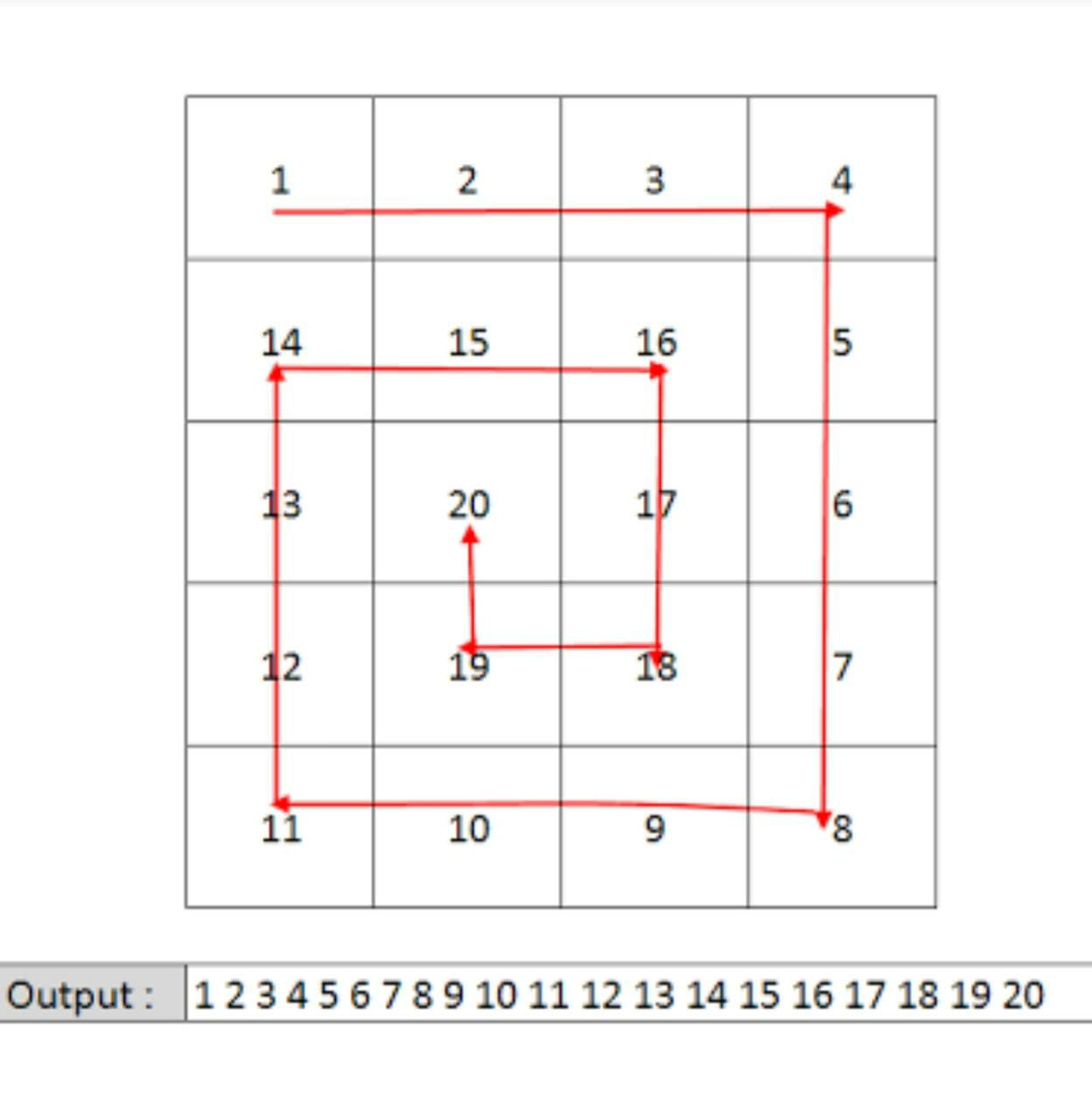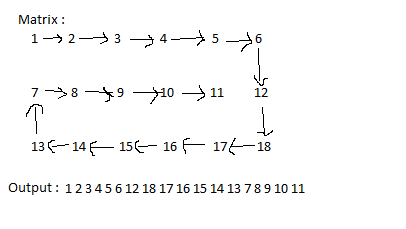11

Spiral Matrix

Difficulty: EASY
Avg. time to solve
15 min
Success Rate
80%

Problem Statement

Example Of Spiral Path:Input Format:
The first line contains an integer 'T' which denotes the number of test cases or queries to be run. Then the test cases follow.

The first line of each test case contains two single space-separated integers 'N' and 'M', denoting the number of rows and columns respectively.

The next 'N' lines, each contains 'M' single space-separated integers representing the elements in a row of the matrix.
Output format :
For each test case/query, print the spiral path of the given matrix.

The output for every test case will be printed in a separate line.
Note:
You do not need to print anything, it has already been taken care of. Just implement the given function.
Constraints:
1 <= T <= 5
1 <= N  <= 10 ^ 2
1 <= M <= 10 ^ 2
-10 ^ 9 <= MATRIX[ i ][ j ] <= 10 ^ 9

Time Limit: 1sec.
Sample Input 1 :
2
4 4
1 2 3 4
5 6 7 8
9 10 11 12
13 14 15 16
3 6
1 2 3 4 5 6
7 8 9 10 11 12
13 14 15 16 17 18
Sample Output 1 :
1 2 3 4 8 12 16 15 14 13 9 5 6 7 11 10
1 2 3 4 5 6 12 18 17 16 15 14 13 7 8 9 10 11
Explanation of the Sample Input 1 :
The spiral path for the test case 2 is as shown below:2
1 1
4
1 5
1 2 3 4 5
4
1 2 3 4 5
Explanation of the Sample Input 2:
In the first test case, there is only one element in the matrix, so the spiral path is only that element.

In the second test case, there is only one row or 1-D matrix, so the spiral path is only the single traversal of the matrix.Console